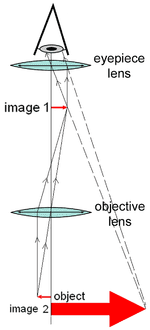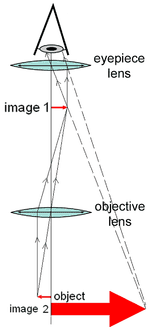# Thin lens

## Homework Statement

The barrel of a compound microscope is 15.9 cm in length. The specimen will be mounted 1.17 cm from the objective, and the eyepiece has a 5.00 cm focal length. Determine the focal length of the objective lens.

## Homework Equations

I am using the mirror equation.

## The Attempt at a Solution

1/1.17 cm+1/s'=1/15.9 cm
-1.26 cm
1/2.433+1/x=1/1.17 this gives me 2.25 cm

I am so lost. Any guidance would be appreciated.[/B]

BvU
Homework Helper
"I am using the mirror equation" is just as lengthy as actually giving that equation. Why not give it ? What mirror equation ?
Why not use a lens equation ? (You do, I think, but it looks weird).

In your working it is unclear what you are doing. Could you be a bit clearer, use symbols and words ?
It looks as if you think the image from the objective is located at the top end of the barrel.
What is -1.26 cm and why do say you add it , but then subtract it ?

I had to search for "mirror" in compound microscope to discover there aren't any (at least not between object and ocular) :)Decide about whether the eye is accomodated or not. Here they say it is focused at 25 cm.

"I am using the mirror equation" is just as lengthy as actually giving that equation. Why not give it ? What mirror equation ?
Why not use a lens equation ? (You do, I think, but it looks weird).

In your working it is unclear what you are doing. Could you be a bit clearer, use symbols and words ?
It looks as if you think the image from the objective is located at the top end of the barrel.
What is -1.26 cm and why do say you add it , but then subtract it ?

I had to search for "mirror" in compound microscope to discover there aren't any (at least not between object and ocular) :)Thanks I figured it out and I meant lens.

Decide about whether the eye is accomodated or not. Here they say it is focused at 25 cm.